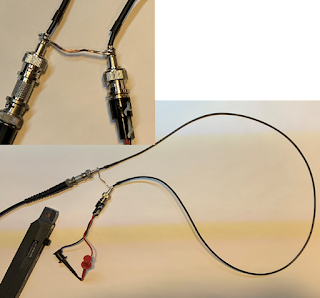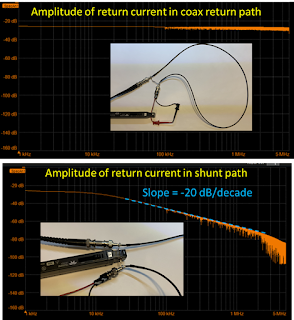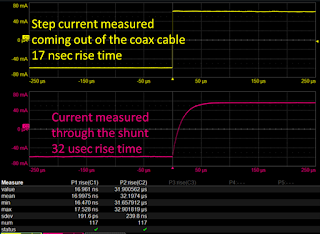## You need to test, we're here to help.

You need to test, we're here to help.

## 18 January 2023

### Return Current at Low Frequency: A Signal and Power Integrity TutorialFigure 3. Specially configured coax cable with the front and back of the shield shorted together.

The following is excerpted from Professor Eric Bogatin's article in the Signal Integrity Journal, The Case for Split Ground Planes. Reprinted by permission of Signal Integrity Journal.

This section continues the discussion in Signal Return Paths of the equation,

Z = R + j𝛚L

Where:

Z is the loop impedance of the current loop path,

R is the series resistance of the loop and

L is the loop inductance of the path.

. . .

At low frequency, when the loop impedance is dominated by the R term, the current distribution in the return plane is NOT driven by the loop impedance, it is driven by the loop resistance. In the signal path, the current will spread out uniformly as any filament path in the signal conductor will have roughly the same resistance.

But the current filaments in the return path with the lowest R will be those which are shortest. This means that return currents will take the shortest paths, independent of the signal paths. As frequency increases, the return current will redistribute to transition from the path of lowest R to the path of lowest L.

This was demonstrated in a simple experiment. A coax cable was shorted at the far end so that a DC current loop, driven by a function generator, would flow from the signal conductor and back through the return. At the front of the coax, the shield, which carries the return current, was shorted between the front of the coax and the back end of the coax. This is shown in Figure 3.Figure 4. Measured return current in theshunt path dropping off above 10 kHz, measured with a Teledyne LeCroy WavePro® HD oscilloscope.
At DC, the return current will flow through the shunt between the front and back of the shield, which is a lower resistance path, instead of flowing all the way down the shield to the far end where the signal and return currents are shorted together.

To measure the current that flows through this path, a Hall effect current clamp was placed around this shunt path. This measures the current flowing through this specific path. The function generator was used to drive a constant 60 mA amplitude of sine wave current through the coax cable and the frequency swept from 1 kHz to 10 MHz. The currents were measured with the Hall effect current probe and a Teledyne LeCroy WavePro HD 12-bit, 8 GHz bandwidth scope.

At low frequency, all the return current flowed through the shunt path. But, as frequency increased, less current flowed through the shunt and more current flowed along the higher resistance, but lower loop inductance path of the coax shield, with the return current in close proximity to the signal current. Figure 4 shows the measured current amplitude in the signal-return loop at the far end, flat with frequency, and the measured current amplitude in the shunt, which drops off with a 1-pole response, above about 10 kHz.Figure 5 Measured step current response through the shunt path with a 10-90 rise time of 32 usec, measured with a Teledyne LeCroy WavePro HD oscilloscope.
This illustrates that above about 10 kHz, all the return current will always flows in the path directly adjacent to the signal current to reduce the loop inductance of the signal-return path. But, equally important, the return currents below about 10 kHz will always flow in the path for lowest resistance.

The transient step response of the current flowing through the shunt path will be a 1-pole response with a pole frequency about 10 kHz. This is an effective RC time constant of about 16 usec. This would result in a 10-90 rise time of about 32 usec. This is what is measured in the step response of the current through the shunt path, shown in Figure 5.